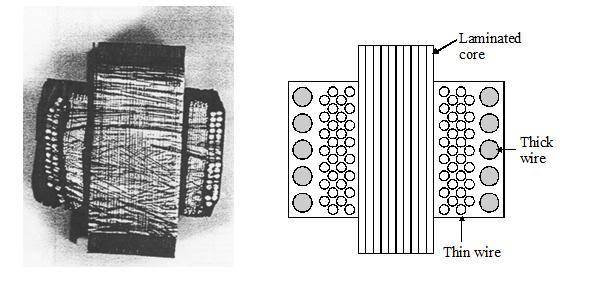# Explain how an e.m.f. is induced in the secondary coil

• Air
The thicker wire is needed to carry the larger current without overheating. The power will be the same on both sides of the transformer (ignoring losses).In summary, the photograph shows a cut transformer with primary and secondary coils wound around the same laminated iron core. One coil has a few turns of thick wire, while the other has many turns of thin wire. The changing current in the primary coil induces an EMF in the secondary coil. When the 512-turn coil is the primary, the transformer functions as a step down by a factor of 16. The 32-turn coil always carries the greater current because of the winding ratio. The thick wire is necessary to carry the larger current without overheating. The laminated core is made

## Homework Statement

The photograph shows a transformer which has been cut in half. The transformer consists of primary and secondary coils wound around the same laminated iron core. The diagram shows a cross-section of the iron core and the two coils. One coil consists of a few turns of thick wire. The other coil has many turns of thinner wire.a) Explain how an e.m.f. is induced in the secondary coil.

b) The transformer in the photograph has one coil with 32 turns of thick wire. and the other with 512 turns of thin wire. Either coil can be the primary. with the other being the secondary. Describe the function of the transformer when the 512-turn coil is the primary.

c) When in use, the 32-turn coil always carries the greater current. Explain why this is so.

d) Explain why this coil is made with much thicker wire.

e) Suggest a reason why the core is made of thin laminated sheets of soft iron.

## Homework Equations

None. This is a theory question.

## The Attempt at a Solution

a) A voltage is provided in the primary coil which produces a current . This changing current causes a magnetic flux in the core. Flux links in the secondary. This changing flux induces a EMF in the secondary coil.

b) Step down by a factor of 16.

c) ?

d) Laminated reduces eddy current. Thin hence reduces it further. Soft iron strengthen the flux.

4. The problem with this question
I don't understand what question (c) is asking for? Can I be guided in the right direction. Thanks in advance.

Last edited:
The power is the same for both primary and secondary coils. You know the transformer winding ratio, right?

Vs/Vp=Ns/Np

From this you can tell that fewer turns will have a lower voltage. To keep the same power the current has to increase.

P=IE

More current means bigger wire.

So, thicker wire provides greater power as the current is large within it?

No the power will be the same on both sides of the transformer (ignoring losses). The thicker wire is needed to carry the larger current without overheating. P=IE or P=IV

Oh, I see. So, a thicker wire is needed so that the same power can be obtained as the thin wire transformer (which has larger turns). The thicker wire are sort of substitution for less turns. Is that correct?

Not really. The thin and thick wire are in the same transformer. The winding of the transformer with the most turns will be at a higher voltage with a lower current. And the winding with the fewest turns will be at a lower voltage with more current.